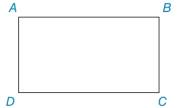Chapter 2.1, Problem 12E### Elementary Geometry for College St...

6th Edition
Daniel C. Alexander + 1 other
ISBN: 9781285195698

#### Solutions

Chapter
Section### Elementary Geometry for College St...

6th Edition
Daniel C. Alexander + 1 other
ISBN: 9781285195698
Textbook Problem
1 views

# A D ¯ ∥ B C ¯ , A B ¯ ∥ D C ¯ , and m ∠ A = 92 ° . Find: a) m ∠ B b) m ∠ C c) m ∠ DTo determine

a)

To find:

The find the measure of B.

Explanation

If there exists two parallel lines cut by a transversal then the pair of interior angles on the same side of transversal are supplementary.

Given:

The given figure is,

The given angle is A.

Approach:

Consider the given figure,

From the above figure, AD¯BC¯ with transversal AB¯

To determine

b)

To find:

The find the measure of C.

To determine

c)

To find:

The find the measure of D.

### Still sussing out bartleby?

Check out a sample textbook solution.

See a sample solution

#### The Solution to Your Study Problems

Bartleby provides explanations to thousands of textbook problems written by our experts, many with advanced degrees!

Get Started

#### 27. Show that the function is a probability density function.

Mathematical Applications for the Management, Life, and Social Sciences

#### Solving Inequalities Solve the inequality. 80. 3 log2 x 4

Precalculus: Mathematics for Calculus (Standalone Book)

#### If a X b, show that a E(X) b.

Probability and Statistics for Engineering and the Sciences

#### let f(x) = x3 + 5, g(x) = x2 2, and h(x) = 2x + 4. Find the rule for each function. 7. fgh

Applied Calculus for the Managerial, Life, and Social Sciences: A Brief Approach

#### The directrix of the conic given by r=62+10sin is: a) x=53 b) x=35 c) y=53 d) y=35

Study Guide for Stewart's Single Variable Calculus: Early Transcendentals, 8th